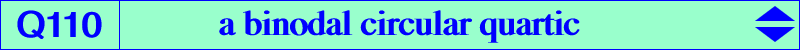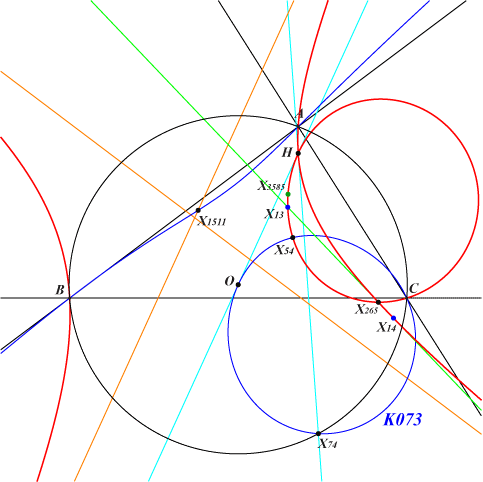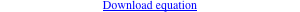too complicated to be written here. Click on the link to download a text file.X(4), X(13), X(14), X(30), X(54), X(265), X(3585)Q110 is related to K073, see property 2 and further details below. This circular quartic has two nodes namely H (with nodal tangents the Euler line and the line passing though X74) and X(265) with complicated nodal tangents. It has two real asymptotes : one is the parallel at X(1511) to the Euler line, the other is complicated. Q110 and K073 With the notations of K073 property 2, denote by La, Lb, Lc the lines A'A", B'B", C'C". It is easy to compute the locus K073 of P such that these lines concur (at Q) but the locus of Q is much more tricky since the equations of the lines have global degree 2 with respect to the coordinates of P. If Q is any point, the line La passes through Q if and only if P lies on a circle Ca. Two other circles Cb, Cc are defined likewise. From this, the lines La, Lb, Lc concur if and only if the circles Ca, Cb, Cc have at least one common point i.e. their Jacobian (J) splits into the line at infinity and a circle with radius 0. It is then sufficient to write that the radius of this circle is 0 to obtain the equation of Q110. Remark : (J) identically vanishes when the circles are in a same pencil which occurs when Q lies on the line through X(4), X(265), etc. In this case, the three circles have two common (finite) points corresponding to the nodes of Q110. If the common points are on the line at infinity, each circle must split into the line at infinity and a line and it can easily be seen that the three lines concur (at X1511) if and only Q = X(30).